Question
in a random sample of four microwave ovens, the mean repair cost was 65.00 and the standard deviation was 12.50 assume the population is normally distributed and use a t-distribution to construct a 95% confidence interval for the population mean u what is the margin of error of u? interpret the results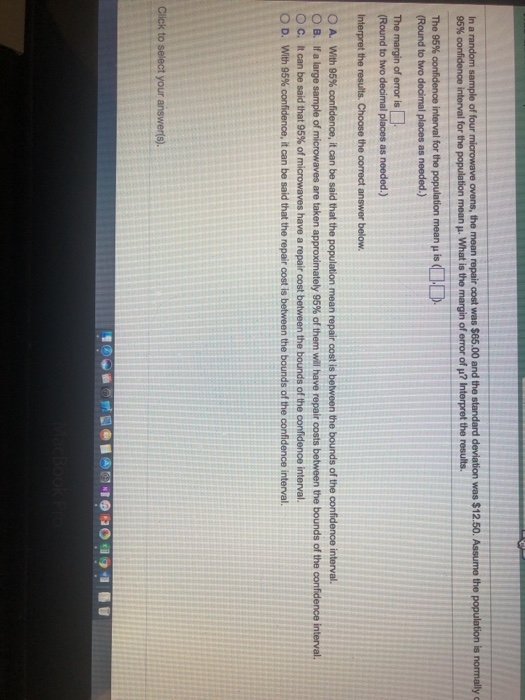Sample size n = 4

Degrees of freedom = n-1= 3

The critical value of t for 3 df with 95% Confidence is +/-3.182

The 95% Confidence Interval for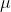is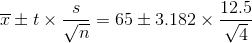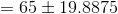The 95% Confidence Interval foris ( 45.11, 84.89)

b) The Margin of Error is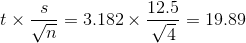c) Interpretation : Option B) If a large sample of microwave are takken approximately 95% of them will have repair costs between the bounds of the confidence interval.

#### Earn Coins

Coins can be redeemed for fabulous gifts.

Similar Homework Help Questions
• ### In a random sample of six microwave​ ovens, the mean repair cost was ​$65.00 and the... In a random sample of six microwave​ ovens, the mean repair cost was ​$65.00 and the standard deviation was ​$12.50. Assume the population is normally distributed and use a​ t-distribution to construct a 90​% confidence interval for the population mean mu. What is the margin of error of mu​? Interpret the results. The 90​% confidence interval for the population mean mu is ​( nothing​, nothing​). ​(Round to two decimal places as​ needed.) The margin of error is nothing. ​(Round to... • ### 6.2.19-T Question Help In a random sample of four microwave ovens, the mean repair cost was...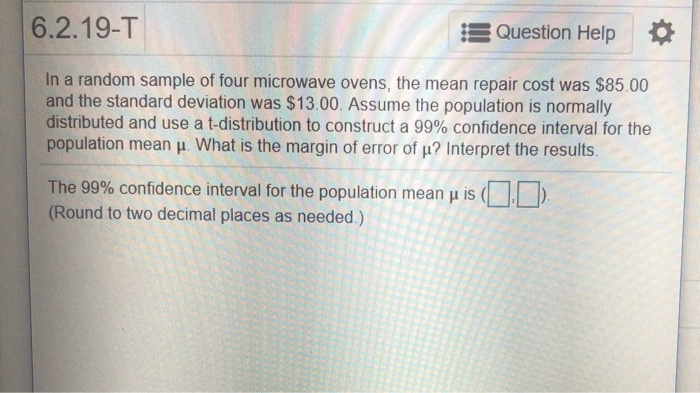6.2.19-T Question Help In a random sample of four microwave ovens, the mean repair cost was$85.00 and the standard deviation was $13.00. Assume the population is normally distributed and use a t-distribution to construct a 99% confidence interval for the population mean μ. What is the margin of error of μ? Interpret the results. The 99% confidence interval for the population mean μ is (DD (Round to two decimal places as needed.) 6.2.21-T Question Help In a random sample... • ### In a random sample of fivefive microwave​ ovens, the mean repair cost was ​$75.0075.00 and the...

In a random sample of fivefive microwave​ ovens, the mean repair cost was ​$75.0075.00 and the standard deviation was ​$14.0014.00. Assume the population is normally distributed and use a​ t-distribution to construct a 9090​% confidence interval for the population mean muμ. What is the margin of error of muμ​? Interpret the results.

• ### Question Help In a random sample of live microwave ovens, the mean repair cost was $60.00...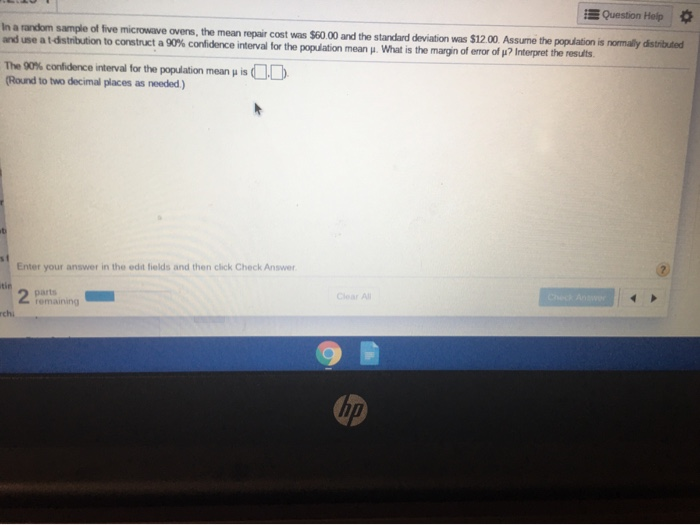Question Help In a random sample of live microwave ovens, the mean repair cost was$60.00 and the standard deviation was $12.00. Assume the population is normally distributed and use at distribution to construct a 90% confidence interval for the population mean . What is the margin of error of ? Interpret the results The 90% confidence interval for the population mean (Round to two decimal places as needed.) is C D Enter your answer in the edit fields and... • ### In a random sample of six microwave? ovens, the mean repair cost was ?$90.00 and the...

In a random sample of six microwave? ovens, the mean repair cost was ?$90.00 and the standard deviation was ?$13.00 Assume the variable is normally distributed and use a? t-distribution to construct a 90?% confidence interval for the population mean ?. What is the margin of error of ???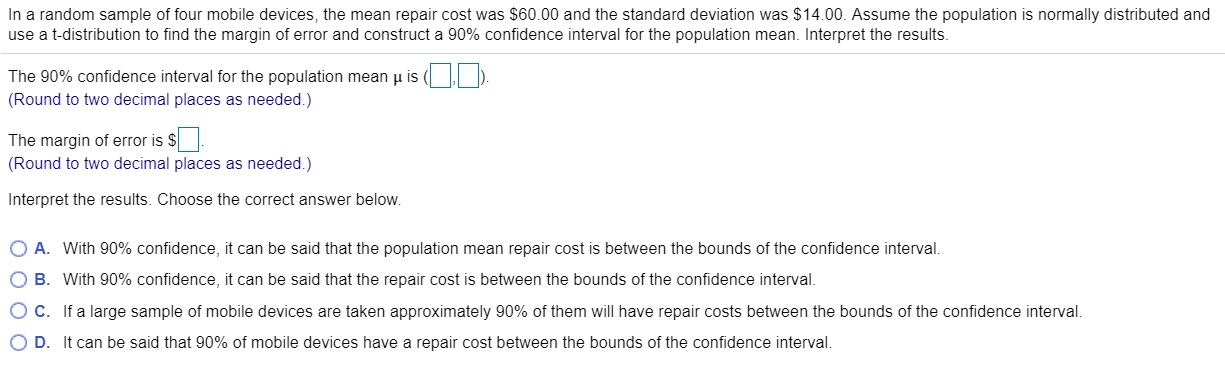In a random sample of four mobile devices, the mean repair cost was $60.00 and the standard deviation was$14.00. Assume the population is normally distributed and use a t-distribution to find the margin of error and construct a 90% confidence interval for the population mean. Interpret the results. The 90% confidence interval for the population mean is (DO (Round to two decimal places as needed.) The margin of error is $(Round to two decimal places as needed.) Interpret... • ### 6.2.20-T Question Help In a random sample of four mobile devices, the mean repair cost was...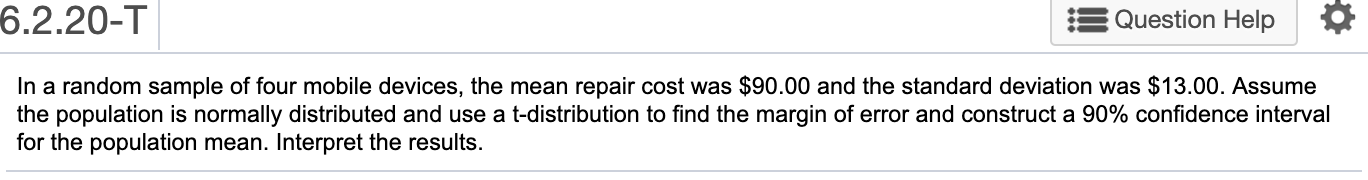6.2.20-T Question Help In a random sample of four mobile devices, the mean repair cost was$90.00 and the standard deviation was $13.00. Assume the population is normally distributed and use a t-distribution to find the margin of error and construct a 90% confidence interval for the population mean. Interpret the results. • ### In a random sample of six mobile devices, the mean repair cost was$70.00 and the...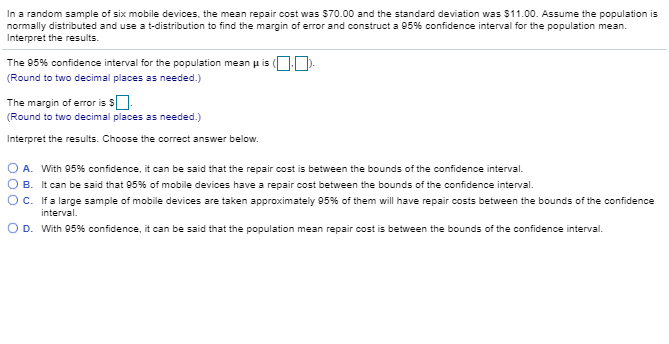In a random sample of six mobile devices, the mean repair cost was $70.00 and the standard deviation was$11.00. Assume the population is normally distributed and use a t-distribution to find the margin of error and construct a 95% confidence interval forte population mean. Interpret the results. The 95% confidence interval for the population m ean μ is (DO). Round to two decimal places as needed.) The margin of error is s (Round to two decimal places as needed.)...